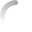# Centripetal Taxation in Action

Want to try your hand at devising a Centripetal Taxation system for the United States? Here's your chance. The object is to maximize the benefits of Centripetal Taxation while making the needed trillion. Then, just for fun, look at your income and see how you would do under your system. For a detailed description of what the parameters mean and how they fit together, see the math. The Differences chart shows how much more—or less—your system makes per tax return than the 2010 average for each category of return defined by the IRS in their tax-return data. It can be viewed either on a linear scale or, since the range can be large, extending in both directions from the x-axis logarithmically (base e). Roll over any chart to see its values; click in the Differences chart to toggle between linear and logarithmic scales, or in either of the line charts to change the c position, and thus the scale (touch devices: move; tap). If you want to share your system, you can copy the generated link.
 t\in [0,1] = \text{the top tax rate} b\in [0,t] = \text{the bottom tax rate} z\in (\text{−}\infty,0) = \text{the zero point} c\in (0,\infty) = \text{the central (ideal) income} m\in [0,\infty) = \text{the basic (minimum) income} f = C =